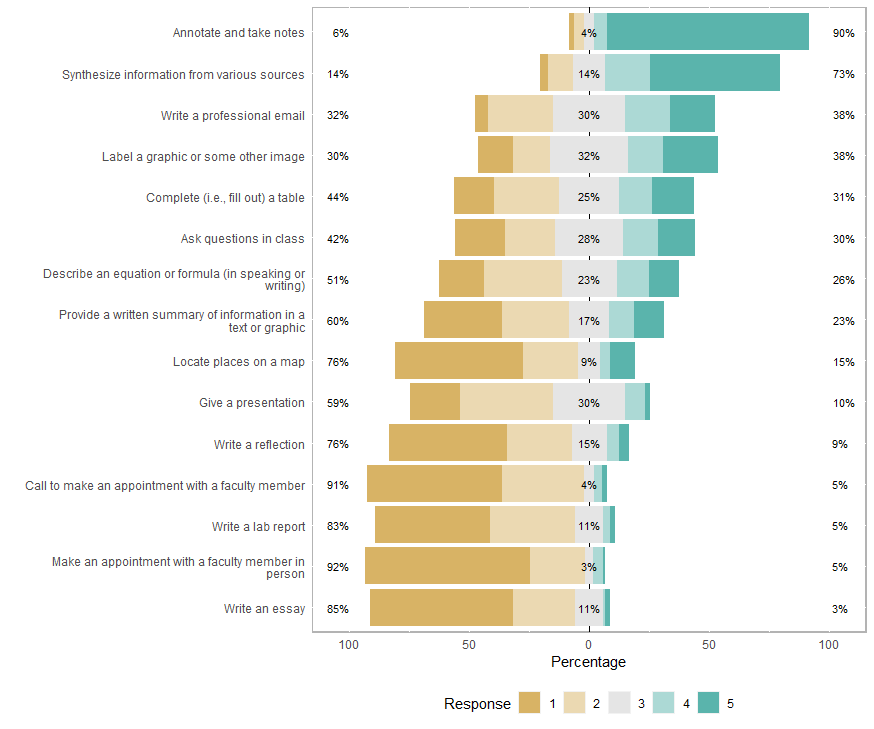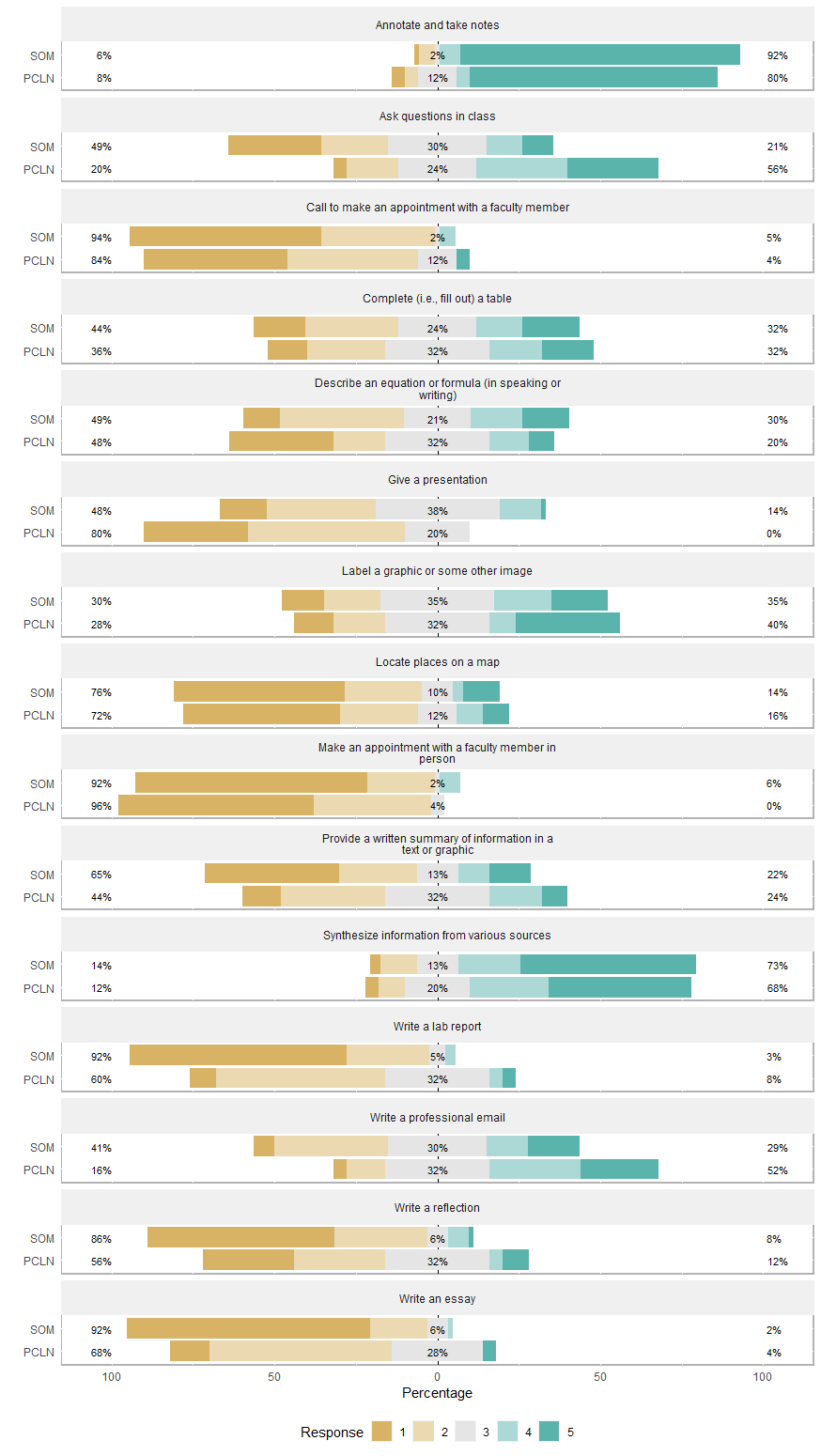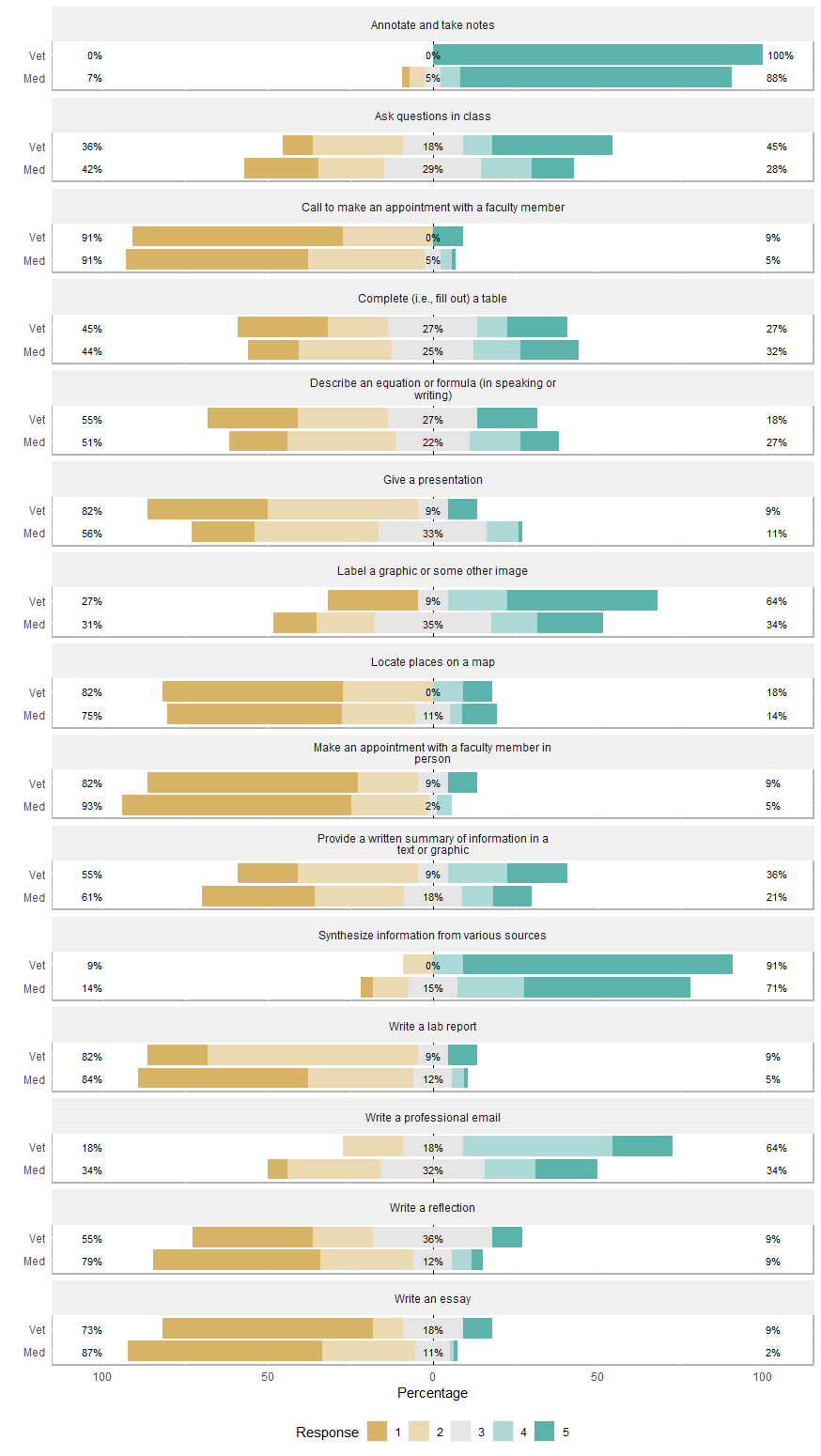There were 15 questions on the questionnaire about different tasks that students have to do. The following figures show a breakdown of students’ responses to the prompt below. Students responded `1 = Never`, `2 = Once a week`, `3 = 2-3 times a week`, `4 = 4-6 times a week`, `5 = Daily`.

``As a student at SGU, how FREQUENTLY do you do the following tasks:``Figure 3.1: Responses for All StudentsFigure 3.2: Responses for Current PCLN vs. SOM StudentsFigure 3.3: Responses for Med vs. Vet Students